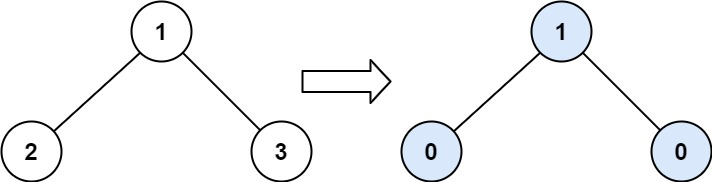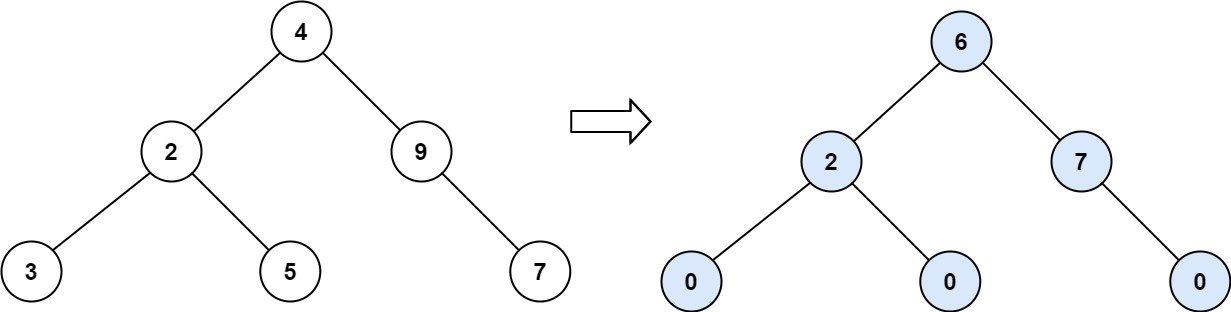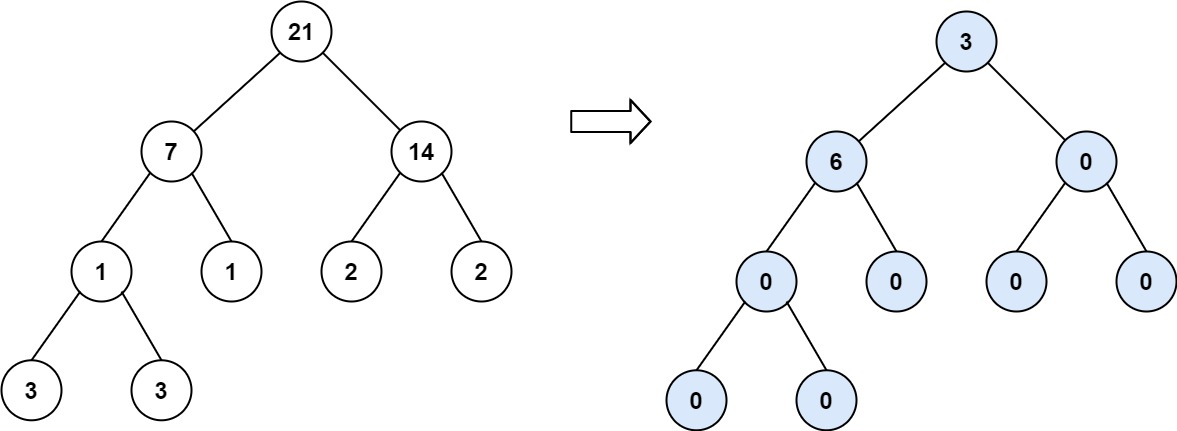### LeetCode• ㊗️
• 大家
• offer
• 多多！

## Problem

Given the root of a binary tree, return the sum of every tree node’s tilt.

The tilt of a tree node is the absolute difference between the sum of all left subtree node values and all right subtree node values. If a node does not have a left child, then the sum of the left subtree node values is treated as 0. The rule is similar if the node does not have a right child.

Example 1:Input: root = [1,2,3]
Output: 1
Explanation:
Tilt of node 2 : |0-0| = 0 (no children)
Tilt of node 3 : |0-0| = 0 (no children)
Tilt of node 1 : |2-3| = 1 (left subtree is just left child, so sum is 2; right subtree is just right child, so sum is 3)
Sum of every tilt : 0 + 0 + 1 = 1


Example 2:Input: root = [4,2,9,3,5,null,7]
Output: 15
Explanation:
Tilt of node 3 : |0-0| = 0 (no children)
Tilt of node 5 : |0-0| = 0 (no children)
Tilt of node 7 : |0-0| = 0 (no children)
Tilt of node 2 : |3-5| = 2 (left subtree is just left child, so sum is 3; right subtree is just right child, so sum is 5)
Tilt of node 9 : |0-7| = 7 (no left child, so sum is 0; right subtree is just right child, so sum is 7)
Tilt of node 4 : |(3+5+2)-(9+7)| = |10-16| = 6 (left subtree values are 3, 5, and 2, which sums to 10; right subtree values are 9 and 7, which sums to 16)
Sum of every tilt : 0 + 0 + 0 + 2 + 7 + 6 = 15



Example 3:Input: root = [21,7,14,1,1,2,2,3,3]
Output: 9


Constraints:

• The number of nodes in the tree is in the range $[0, 10^4]$.
• -1000 <= Node.val <= 1000

## Code

class Solution {
int res = 0;

public int findTilt(TreeNode root) {
helper(root);
return res;
}

private int helper(TreeNode root) {
if(root == null) return 0;

int left = helper(root.left);
int right = helper(root.right);

res +=  Math.abs(left - right);

return root.val + left + right;
}
}